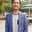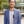Trusted answers to developer questions
Trusted Answers to Developer Questions

Related Tags

python

# What is the __str__ method in Python?Shahpar Khan

Grokking Modern System Design Interview for Engineers & Managers

Ace your System Design Interview and take your career to the next level. Learn to handle the design of applications like Netflix, Quora, Facebook, Uber, and many more in a 45-min interview. Learn the RESHADED framework for architecting web-scale applications by determining requirements, constraints, and assumptions before diving into a step-by-step design process.## The need for __str__ method:

The __str__ method in Python represents the class objects as a string – it can be used for classes. The __str__ method should be defined in a way that is easy to read and outputs all the members of the class. This method is also used as a debugging tool when the members of a class need to be checked.

The __str__ method is called when the following functions are invoked on the object and return a string:

• print()
• str()

If we have not defined the __str__, then it will call the __repr__ method. The __repr__ method returns a string that describes the pointer of the object by default (if the programmer does not define it).

## How to call __str__ method

### 1. Default implementation

class MyClass:    x = 0    y = ""    def __init__(self, anyNumber, anyString):        self.x = anyNumber        self.y = anyStringmyObject = MyClass(12345, "Hello")print(myObject.__str__())print(myObject.__repr__())print(myObject)

The above code shows an example where neither __str__ nor __repr__ are defined. Calling __str__ calls the default __repr__ method, and they all give the same output, the pointer of our object.

### 2. Custom __str__ method

class MyClass:    x = 0    y = ""    def __init__(self, anyNumber, anyString):        self.x = anyNumber        self.y = anyString    def __str__ (self):        return 'MyClass(x=' + str(self.x) + ' ,y=' + self.y + ')'myObject = MyClass(12345, "Hello")print(myObject.__str__())print(myObject)print(str(myObject))print(myObject.__repr__())

The code above shows the output once you have defined the __str__ method. When __str__, print(), or str() are called you will get your defined output. Make note that the __repr__ output remains the same.

### 3. __repr__ method defined only

class MyClass:    x = 0    y = ""    def __init__(self, anyNumber, anyString):        self.x = anyNumber        self.y = anyString    def __repr__ (self):        return 'MyClass(x=' + str(self.x) + ' ,y=' + self.y + ')'myObject = MyClass(12345, "Hello")print(myObject.__str__())print(myObject)print(str(myObject))print(myObject.__repr__())

In the first example we saw that when __str__ is not defined it automatically calls the __repr__ method. Therefore, the output of all the functions - __str__, str(), and __repr__ - are the same. Moreover, the __repr__ method does not necessarily need to return a string. In case it does not return a string, the print() statements will throw an error.

RELATED TAGS

python

CONTRIBUTORShahpar Khan